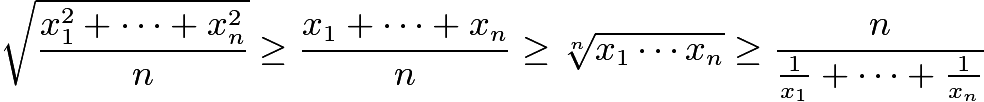# Recursively defined numbers, need to show they are non-decreasing

Hello, I have a question involving a sequence of numbers $$\{a_n\}$$ defined recursively. They are defined as the positive solutions of the following set of equations.

$$1 = \frac{1}{a_1} = \frac{1}{(a_2+a_1)} + \frac{1}{{a_2}} = \frac{1}{(a_3 + a_2 +a_1)} + \frac{1}{(a_3 + a_2)} + \frac{1}{{a_3}} = \cdots = \frac{1}{(a_n + a_{n-1}+ \cdots +a_1)} + \frac{1}{(a_n + a_{n-1}+ \cdots +a_2)} + \cdots + \frac{1}{{a_n}} = \cdots$$

The first few can be solved analytically $$a_1 = 1, a_2 \approx 1.62, \cdots$$. The rest can be found numerically.

However, instead of their values, what I need is to show that these numbers have to be non-decreasing, i.e. $$a_{n+1} \geq a_{n}$$ for all n.

Any ideas welcome.

Thank you.

Notes:

An observation that might be helpful is that, the numerical solutions show a_n's grow with natural logarithm. ($$\ln{(2n+2)}$$ seems to fit the numerical solution perfectly. ) Also, $$a_n \geq H_n, \text{\, where \,} H_n = \sum_{k=1}^{n}\frac{1}{k} \text{\, is the n^{\text{th}} harmonic number.}$$

The numerical solution suggests $$a_{n+1} - a_n$$ goes to zero as n goes to infinity. So this might suggest induction is the way to go for the proof. Assume non-decreasing up to a_n, show that $$a_{n+1} \geq a_{n}$$.

Last edited: